﻿ New Unified Integral Involving General Polynomials of Multivariable H-function

### New Unified Integral Involving General Polynomials of Multivariable H-function

Neelam Pandey, Ashiq Hussain Khan

## New Unified Integral Involving General Polynomials of Multivariable H-function

Neelam Pandey1, Ashiq Hussain Khan1,1Department of Mathematics Govt. Girl’s P. G. College Rewa (M. P.), India

### Abstract

In the present paper, the author establish new unified integral whose integral contains products of H-function of several complex variable  and a general polynomials given by Srivastava  with general arguments. A large number of integrals involving various simpler functions follow as special cases of this integral.

• Neelam Pandey, Ashiq Hussain Khan. New Unified Integral Involving General Polynomials of Multivariable H-function. American Journal of Applied Mathematics and Statistics. Vol. 5, No. 1, 2017, pp 11-13. http://pubs.sciepub.com/ajams/5/1/3
• Pandey, Neelam, and Ashiq Hussain Khan. "New Unified Integral Involving General Polynomials of Multivariable H-function." American Journal of Applied Mathematics and Statistics 5.1 (2017): 11-13.
• Pandey, N. , & Khan, A. H. (2017). New Unified Integral Involving General Polynomials of Multivariable H-function. American Journal of Applied Mathematics and Statistics, 5(1), 11-13.
• Pandey, Neelam, and Ashiq Hussain Khan. "New Unified Integral Involving General Polynomials of Multivariable H-function." American Journal of Applied Mathematics and Statistics 5, no. 1 (2017): 11-13.

 Import into BibTeX Import into EndNote Import into RefMan Import into RefWorks

### 1. Introduction

The H-function of several complex variables is defined by Srivastava and Panda  as: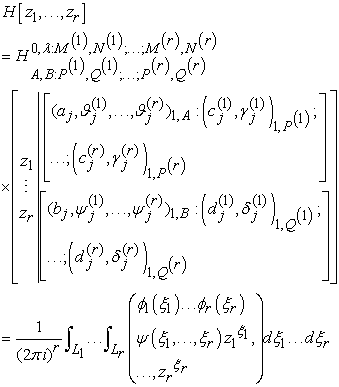(1.1)

where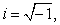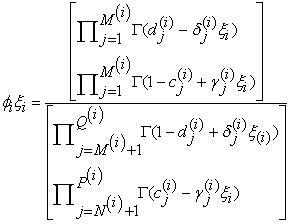(1.2)

for all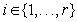and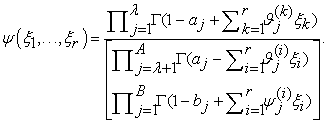(1.3)

The H-function of several complex variables in (1.1) converges absolutely if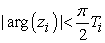(1.4)

where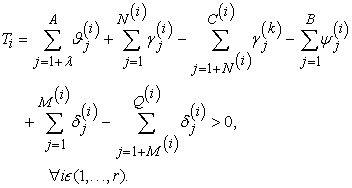(1.5)

The general polynomials have been defined and introduced by Srivastava  as following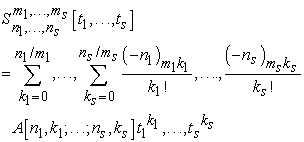(1.6)

where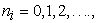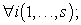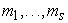arbitrary positive integers and the coefficient are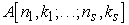are arbitrary constants, real or complex.

### 2. Main Result

In this section, we have derived the following integral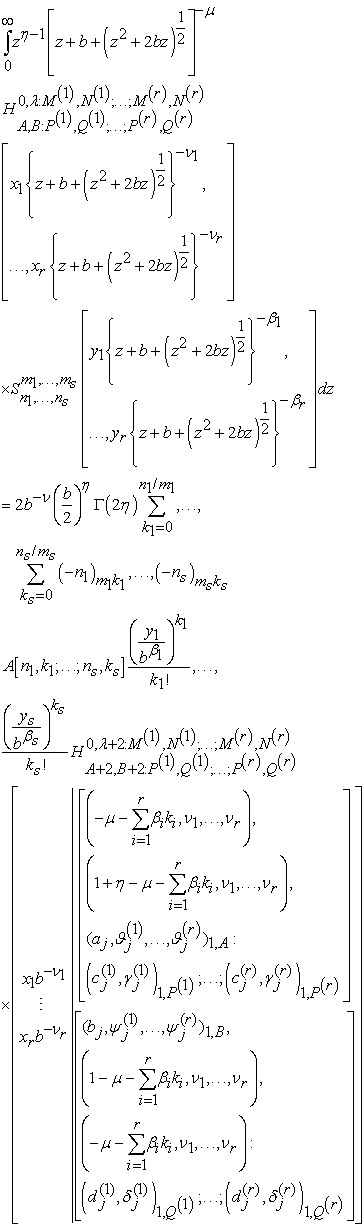(2.1)

provided that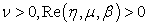and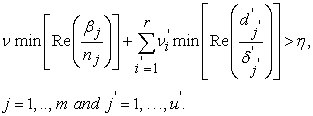Proof: In obtain result (2.1), first we express the H-function of several complex variables in terms of Mellin-Barnes contour integrals using eq. (1.1) and the general polynomial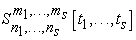in series from given by eq. (1.6). Now interchanging the order of summation and integration which is permissible under the stated conditions, we obtain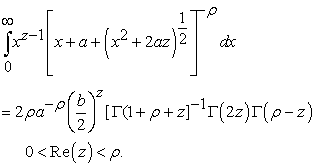(2.2)

Evaluating the above z-integral with the help of a known result given  and reinterpreting the result thus obtained in terms of H-function of r-variables, we reach at the desired result.

### 3. Special Cases

I. Taking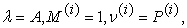and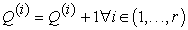the result in (2.1) reduces to the following integral transformation: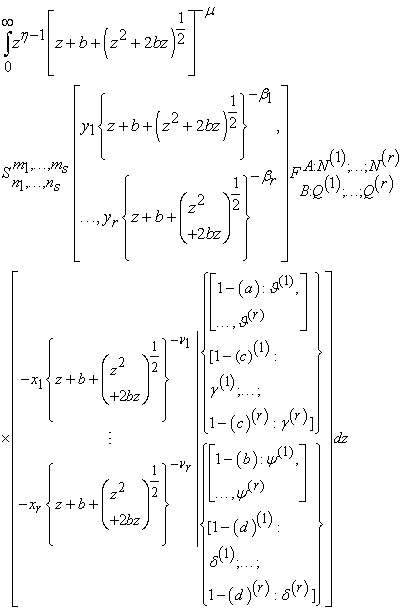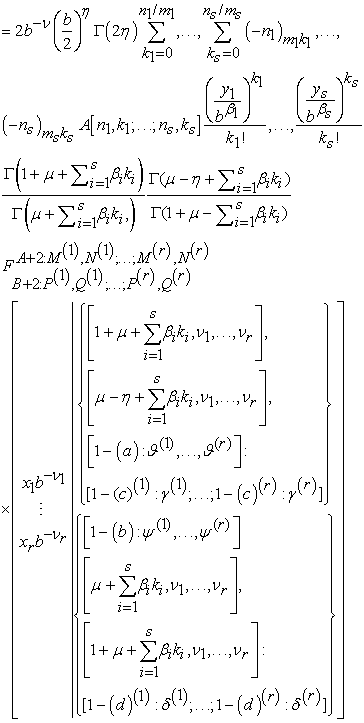II. When we put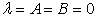in (2.1) we get the following transformation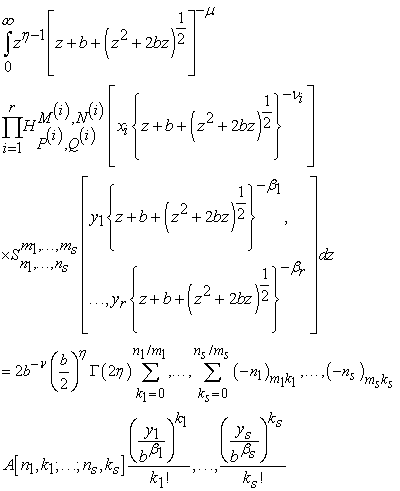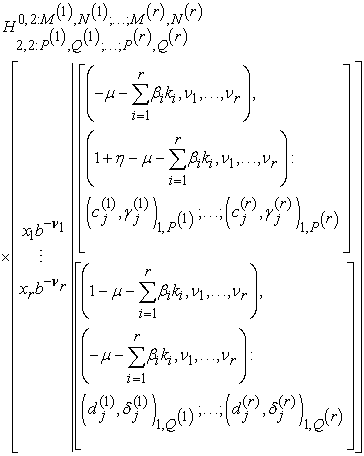III. If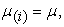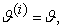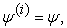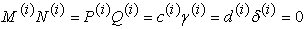and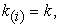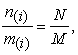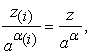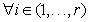the result in (2.1) reduces to the known result with a small modification derived by Garg and Mittal .

### 4. Conclusion

Finally we conclude with the remark that results and the operators proved in this paper appear to be new and likely to have useful applications to a wide range of problems of mathematics, statistics and physical sciences.

### References

  H. M. Srivastava and R. Panda, Some bilateral generating function for a class of generalized hypergeometric polynomials, J. Raine Angew. Math 283/284 (1996), 265-274.In article  H. M. Srivastava, A multilinear generating function for the konhauser sets of bi-orthogonal polynomials suggested by the Laguerre polynomials, Pacific J. Math. 117 (1985) 183-191.In article View Article  H. M. Srivastava, A contour integral involving Fox’s H-function, India J. Math. 14 (1972) 1-6.In article  F. Oberhettinger, Tables of Mellin transforms (Berlin, Heidelberg, New York: Springer-Verlag) (1974) p.22.In article  H. M. Srivastava, K. C. Gupta and S. P. Goyal, the H-function of one and two variables with applications (New Delhi and Madras: South Asian Publ.) (1982) p. 11, 18-19.In article  Mrigula Gang and Shweta Mittal, on a new unified integral, Proc. India Acad. Sci. (Math. Sci.) vol. 114, 2 (2004), pp. 99-101.In article